Next: The vector product Up: Rotational motion Previous: Rigid body rotation

## Is rotation a vector?

Consider a rigid body which rotates through an angle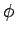about a given axis. It is tempting to try to define a rotation vector''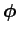which describes this motion. For example, suppose thatis defined as the vector'' whose magnitude is the angle of rotation,, and whose direction runs parallel to the axis of rotation. Unfortunately, this definition is ambiguous, since there are two possible directions which run parallel to the rotation axis. However, we can resolve this problem by adopting the following convention--the rotation vector'' runs parallel to the axis of rotation in the sense indicated by the thumb of the right-hand, when the fingers of this hand circulate around the axis in the direction of rotation. This convention is known as the right-hand grip rule. The right-hand grip rule is illustrated in Fig. 68.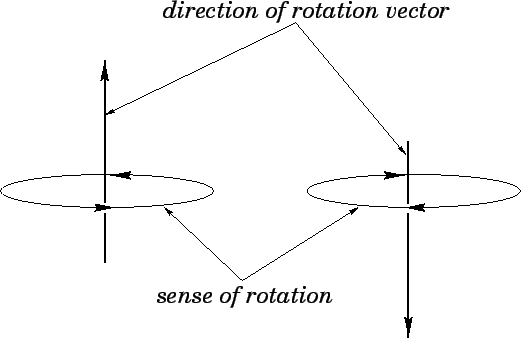The rotation vector''now has a well-defined magnitude and direction. But, is this quantity really a vector? This may seem like a strange question to ask, but it turns out that not all quantities which have well-defined magnitudes and directions are necessarily vectors. Let us review some properties of vectors. If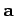and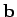are two general vectors, then it is certainly the case that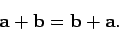(315)

In other words, the addition of vectors is necessarily commutative (i.e., it is independent of the order of addition). Is this true for vector'' rotations, as we have just defined them? Figure 69 shows the effect of applying two successiverotations--one about the-axis, and the other about the-axis--to a six-sided die. In the left-hand case, the-rotation is applied before the-rotation, and vice versa in the right-hand case. It can be seen that the die ends up in two completely different states. Clearly, the-rotation plus the-rotation does not equal the-rotation plus the-rotation. This non-commutative algebra cannot be represented by vectors. We conclude that, although rotations have well-defined magnitudes and directions, they are not, in general, vector quantities.

There is a direct analogy between rotation and motion over the Earth's surface. After all, the motion of a pointer along the Earth's equator from longitudeW to longitudeW could just as well be achieved by keeping the pointer fixed and rotating the Earth throughabout a North-South axis. The non-commutative nature of rotation vectors'' is a direct consequence of the non-planar (i.e., curved) nature of the Earth's surface. For instance, suppose we start off at (N,W), which is just off the Atlantic coast of equatorial Africa, and rotatenorthwards and theneastwards. We end up at (N,E), which is in the middle of the Indian Ocean. However, if we start at the same point, and rotateeastwards and thennorthwards, we end up at the North pole. Hence, large rotations over the Earth's surface do not commute. Let us now repeat this experiment on a far smaller scale. Suppose that we walk 10m northwards and then 10m eastwards. Next, suppose that--starting from the same initial position--we walk 10m eastwards and then 10m northwards. In this case, few people would need much convincing that the two end points are essentially identical. The crucial point is that for sufficiently small displacements the Earth's surface is approximately planar, and vector displacements on a plane surface commute with one another. This observation immediately suggests that rotation vectors'' which correspond to rotations through small angles must also commute with one another. In other words, although the quantity, defined above, is not a true vector, the infinitesimal quantity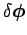, which is defined in a similar manner but corresponds to a rotation through an infinitesimal angle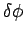, is a perfectly good vector.

We have just established that it is possible to define a true vectorwhich describes a rotation through a small angleabout a fixed axis. But, how is this definition useful? Well, suppose that vectordescribes the small rotation that a given object executes in the infinitesimal time interval betweenand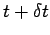. We can then define the quantity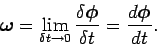(316)

This quantity is clearly a true vector, since it is simply the ratio of a true vector and a scalar. Of course,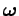represents an angular velocity vector. The magnitude of this vector,, specifies the instantaneous angular velocity of the object, whereas the direction of the vector indicates the axis of rotation. The sense of rotation is given by the right-hand grip rule: if the thumb of the right-hand points along the direction of the vector, then the fingers of the right-hand indicate the sense of rotation. We conclude that, although rotation can only be thought of as a vector quantity under certain very special circumstances, we can safely treat angular velocity as a vector quantity under all circumstances.

Suppose, for example, that a rigid body rotates at constant angular velocity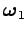. Let us now combine this motion with rotation about a different axis at constant angular velocity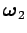. What is the subsequent motion of the body? Since we know that angular velocity is a vector, we can be certain that the combined motion simply corresponds to rotation about a third axis at constant angular velocity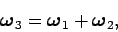(317)

where the sum is performed according to the standard rules of vector addition. [Note, however, the following important proviso. In order for Eq. (317) to be valid, the rotation axes corresponding toandmust cross at a certain point--the rotation axis corresponding to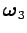then passes through this point.] Moreover, a constant angular velocity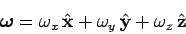(318)

can be thought of as representing rotation about the-axis at angular velocity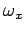, combined with rotation about the-axis at angular velocity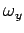, combined with rotation about the-axis at angular velocity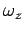. [There is, again, a proviso--namely, that the rotation axis corresponding tomust pass through the origin. Of course, we can always shift the origin such that this is the case.] Clearly, the knowledge that angular velocity is vector quantity can be extremely useful.Next: The vector product Up: Rotational motion Previous: Rigid body rotation
Richard Fitzpatrick 2006-02-02